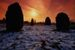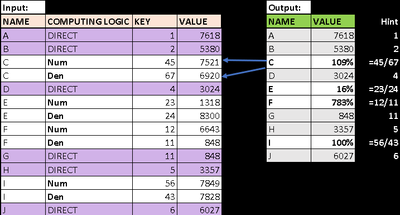Announcements
Applications are open for the 2024 Qlik Luminary Program. Apply by December 15 here.
cancel
Showing results for
Did you mean:Creator

## SET EXPRESSION LOGIC

Hi all,

I have a table script which has 2 different computation logic:

1. Direct: Here, I can get values for respective names

2. Division: Names' value need to be calculated by dividing keys, here, I am getting issues.

Kindly suggest how to proceed here. Note: Script can't be amended.

Below is the sample input table & desired output:Note: For ease I have shown values column in input though it is in fact table.

Thank you.

Labels (1)
• ### General Question

1 Solution

Accepted SolutionsPartner - Creator

Hello,

``IF(COMP_LOGIC='DIVISION', NUM(AGGR(ABOVE(VALUE),COMP_LOGIC,NAME,VALUE)/AGGR(BELOW(VALUE),COMP_LOGIC,NAME,VALUE),'###%'),VALUE)``2 RepliesPartner - Creator

Hello,

``IF(COMP_LOGIC='DIVISION', NUM(AGGR(ABOVE(VALUE),COMP_LOGIC,NAME,VALUE)/AGGR(BELOW(VALUE),COMP_LOGIC,NAME,VALUE),'###%'),VALUE)``Creator
Author

Thanks @ckarras22 , no doubt above expression is working as expected to the dataset given however just an amd required (which is in point 2).

1.  My "value" column is a set expression, still they will work inside agg(above(sum({\$<conditions>}Values)….

2. The dataset actually has Num,Denom values instead of division (as I was thinking "Key" column is more imp), refer below:3. Query: Name F is  a outcome of keys calculation ie 12/11 & Name G itself is key 11. So,  I think calculation made using keys will be more helpful,  what are your thoughts?

Thank you.Tags
Community Browser